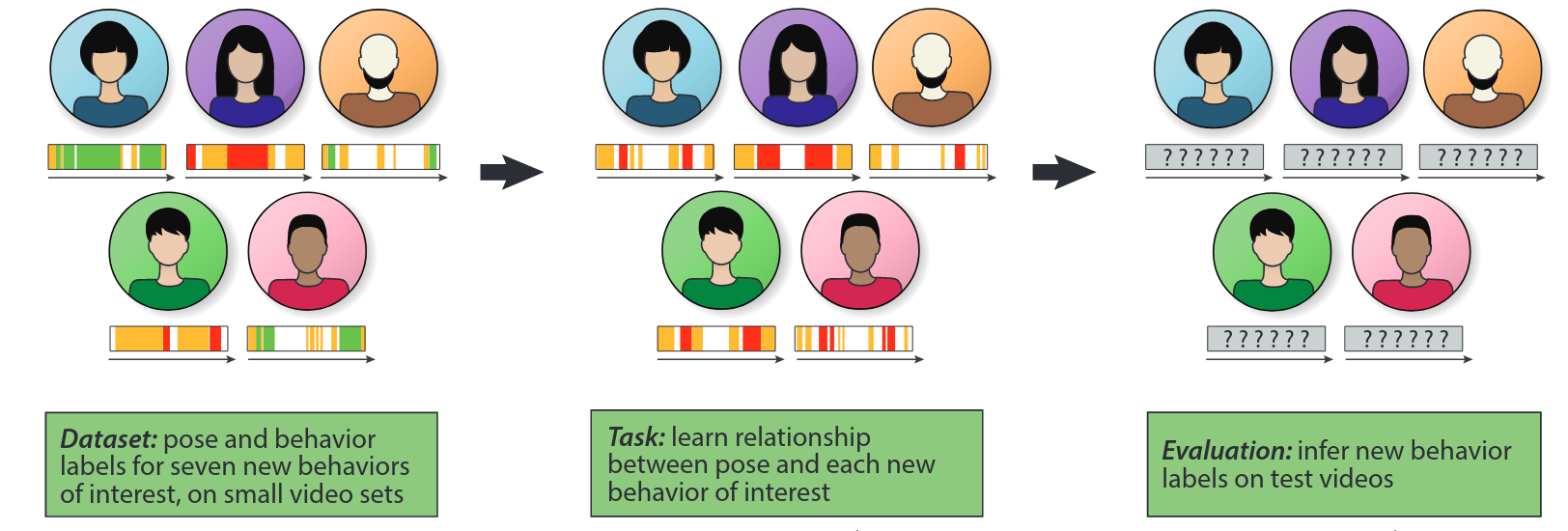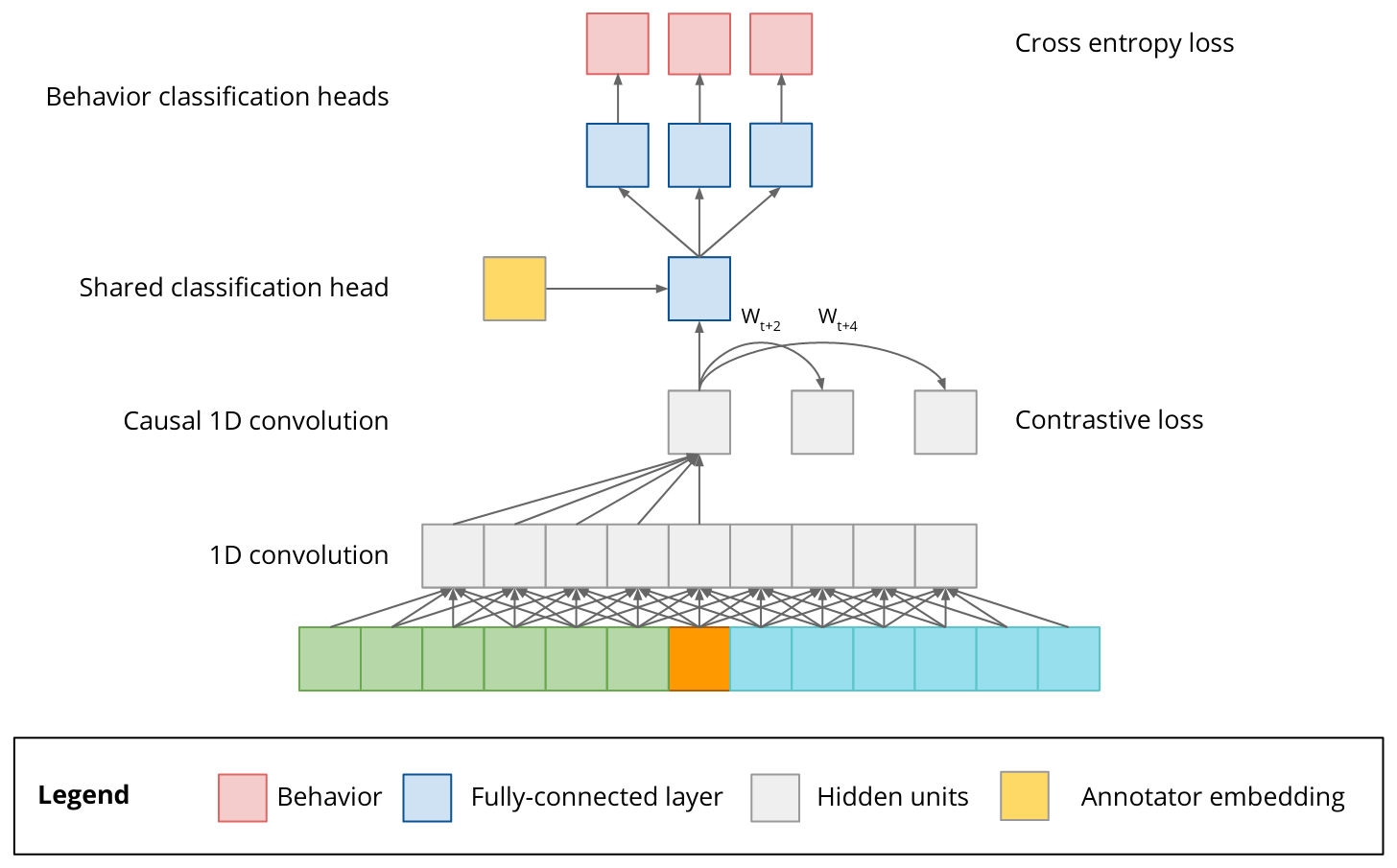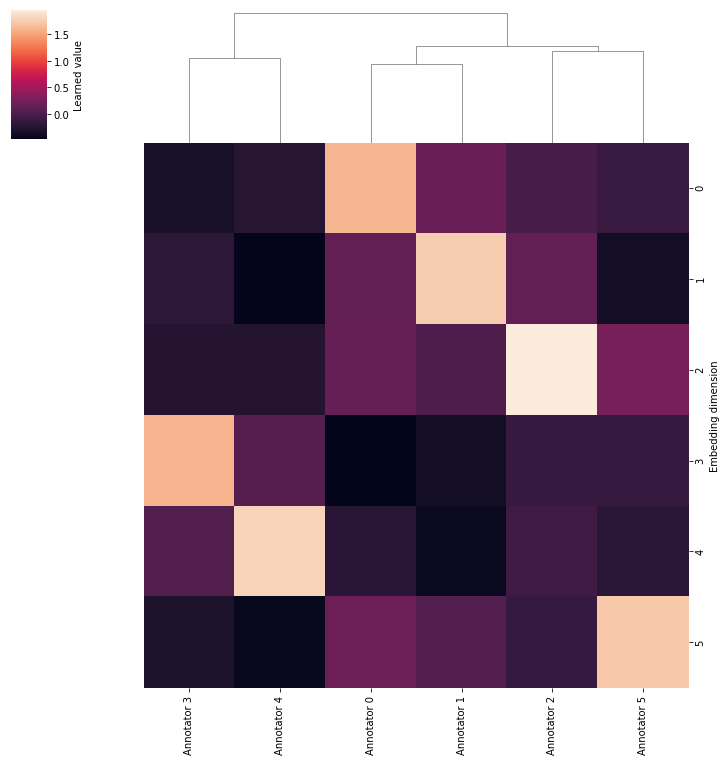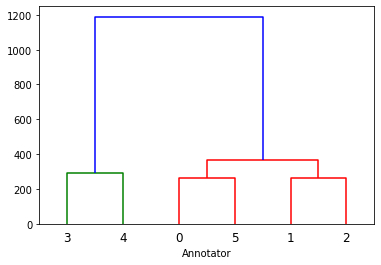# Clustering of learned annotator embeddings

Using the task 1 and 2 winning solution model to cluster annotation styles from task 2.

In this notebook, we will use the winning model for task 1 and 2 to cluster the annotation style of the different annotators from task 2.
We will use a pretrained model and precomputed predictions for all frames from the test videos.
Clustering the learned annotation embeddings from the pretrained model reveals two high-level clusters of annotation styles.
Finally, we compare the annotations embeddings and corresponding clusters to directly clustering the model predictions.

# Clustering of learned annotator embeddings¶

In this notebook, we will use the winning model for task 1 and 2 to cluster the annotation style of the different annotators from task 2.

You can learn more about the model in the paper preprint and in the solution outline in the github repository.# Install dependencies¶

First of all, we need to install the dependencies, which in this case are simply PyTorch and the module containing all the code from my solution.

In [ ]:
!pip install torch
!pip install git+https://github.com/nebw/mabe.git


Training the model requires a lot of time and compute, and the training code assumes that the training data has already been preprocessed.

Fortunately, we can completely skip the model training for our purposes and load a file with the pretrained model parameters and predictions for all test videos.

The training results are quite large, and we will load them from a shared Google Drive:

In :
from pydrive.auth import GoogleAuth

auth.authenticate_user()

file_id = '1X7RGaoBtFwIvedfoAioZ8Ft1d7PvxbP0'



Here we load the TrainingResult object that we just downloaded and instantiate a MultiAnnotatorLogisticRegressionHead, the final component in the model architecture.The MultiAnnotatorLogisticRegressionHead consists of a LayerNorm layer, a residual block, and final linear layers for each classification from Task 1 and 3 (i.e., a multinomial classification layer for Task 1, and 7 binary classification layers for Task 3). The residual block has one additional input: A learned embedding of the annotator for Task 3. This embedding is initialized as a diagonal matrix, i.e. the embedding for annotator 0 will initially be $[1, 0, 0, 0, 0, 0]$. The model can then learn similar embeddings for annotators with a similar style and the residual block can modify the inputs to the classification head to match the style of each annotator. The annotator embeddings are kept small to avoid overfitting, but I did not experiment with larger or different kinds of embeddings.

In :
import torch
import numpy as np
import pandas as pd

import mabe
import mabe.model

In :
training_result = torch.load('pretrained.pt')

In :
# usually we would load these hyperparameters directly from the data, but here
# we define them manually to avoid having to download and preprocess the
# training data. they can be ignored in the context of this notebook.
num_extra_features = 4

num_annotators = 6
annotators = np.arange(num_annotators)

In :
# instantiate a new MultiAnnotatorLogisticRegressionHead object
training_result.config.num_context,
num_annotators,
num_extra_features,
)

In :
def load_annotator_embeddings(logreg):
# load pretrained annotator embeddings from model and store them in a pandas
# dataframe for convenient analysis
annotator_embedding = logreg.embedding.cpu().data.numpy().T
annotator_embedding = pd.DataFrame(
annotator_embedding, columns=[f"Annotator {a}" for a in range(6)]
)
return annotator_embedding

In :
load_annotator_embeddings(logreg)

Out:
Annotator 0 Annotator 1 Annotator 2 Annotator 3 Annotator 4 Annotator 5
0 1.0 0.0 0.0 0.0 0.0 0.0
1 0.0 1.0 0.0 0.0 0.0 0.0
2 0.0 0.0 1.0 0.0 0.0 0.0
3 0.0 0.0 0.0 1.0 0.0 0.0
4 0.0 0.0 0.0 0.0 1.0 0.0
5 0.0 0.0 0.0 0.0 0.0 1.0

We can see that the annotator embeddings for a new instance of the logistic regression hat are simply a diagonal matrix.

In :
# load parameters from epoch with best validation loss during training
cpc_state_dict, logreg_state_dict = training_result.best_params
_ = logreg.eval()


We can now load the learned annotator embeddings from the pretrained model and cluster them.

In :
annotator_embedding = load_annotator_embeddings(logreg)
annotator_embedding

Out:
Annotator 0 Annotator 1 Annotator 2 Annotator 3 Annotator 4 Annotator 5
0 1.615375 0.172890 -0.028071 -0.328272 -0.229189 -0.115436
1 0.142648 1.763415 0.125250 -0.192525 -0.473131 -0.364142
2 0.158884 0.011661 1.955692 -0.252963 -0.251466 0.253844
3 -0.486687 -0.367095 -0.133002 1.600027 0.057513 -0.137715
4 -0.223837 -0.425962 -0.082107 0.029151 1.802917 -0.210606
5 0.183608 0.030458 -0.134308 -0.304217 -0.432414 1.727410

The annotator embeddings have changed significantely during training, but what does that tell us about the annotation styles of the different annotators?

# Clustering of annotator embeddings¶

The annotator embeddings are initialized as a diagonal matrix, but we expect the model to learn similar embeddings for two annotators if they share a similar annotation style.

We can quantify this by computing the pairwise euclidean distances between the embeddings of the all annotators and then perform hierachical clustering using these distances.

Fortunately, we can use the clustermap function provided by seaborn to perform the distance calculation, clustering, and visualization automatically.

In :
import io
import seaborn as sns
import scipy
import scipy.special
import scipy.spatial as sp
import scipy.cluster.hierarchy as hc
import matplotlib.pyplot as plt

In :
# perform and plot hierachical clustering on the annotator embeddings. similar
# embeddings (with a low euclidean distance) will be assigned to the same
# cluster
cm = sns.clustermap(
annotator_embedding, row_cluster=False, method="ward",
cbar_kws={"label": "Learned value"}
)
cm.ax_heatmap.set_ylabel("Embedding dimension")
plt.setp(cm.ax_heatmap.get_xticklabels(), rotation=90)

Out:
[None, None, None, None, None, None, None, None, None, None, None, None]We can see that the hierachical clustering has assigned two high level clusters to the annotator embeddings. It appears that Annotator 3 and 4 share a similar style. The other annotators get assigned to the second cluster, but there also appears to be some structure here, with Annotator 0 and 1 and Annotator 2 and 5 forming two subclusters.

# Clustering of model predictions¶

We have to be careful when interpreting these results, though. We can't safely assume that low euclidean distances in the annotator embeddings are equivalent with similar annotation styles.

The annotator embeddings are used in the final component of the model, but there are still several fully connected layers with nonlinearities in this final component.

We can verify our previous results by clustering the predictions of to model for the different annotators.

The TrainingResults object we've loaded above already contains the predictions of the model for all test videos and all annotators.

In :
# sequence IDs from all test videos
sequences = list(training_result.test_predictions.keys())


Due to the size of the predictions, we now have to calculate the distance matrix manually.

The model predictions are stored as compressed numpy arrays, so we need a bit of boilerplate code to load them:

In :
distances = []

# iterate over all test video sequence IDs
for sequence_id in sequences:
# this will store the probabilites the model assigns to each class for each
# frame, for each annotator
predictions_by_annotator = []

# iterate over all annotators
for annotator_id in annotators:
# the results are stored as compressed numpy arrays, so we need to load
# them into a BytesIO object first s.t. numpy.load can read them like a
# normal file
compressed_results = io.BytesIO(
training_result.test_logits[sequence_id][annotator_id]
)

# we can now load the model predictions for the current video and
# annotator. We apply the softmax function to turn the model outputs
# (logits) to probabilities
predictions = scipy.special.softmax(logits, -1)

predictions_by_annotator.append(predictions)

# we can now stack and flatten the predictions for each annotator
predictions_by_annotator = np.stack(predictions_by_annotator)
predictions_flat = predictions_by_annotator.reshape(num_annotators, -1)

# loaded all predictions from all videos at once and calculating the distance
# matrix in one step would be quite memory intensive, so we simply calculate
# the distance matrix for each video seperately and sum them up at the end.
# note that pdist returns a condensed distance matrix which works out fine
# in this case, because that's the format that scipy's linkage function
# expects anyways.
sequence_distances = scipy.spatial.distance.pdist(
predictions_flat,
lambda u, v: ((u - v) ** 2).sum()
)

distances.append(sequence_distances)

distances = np.stack(distances)
# sum up the precomputed distance matrices and apply sqrt to get the final
# euclidean distance matrix.
distances = np.sqrt(np.sum(distances, axis=0))

In :
# finally, we can use scipy to perform and visualize the hierachical clustering.
dn = scipy.cluster.hierarchy.dendrogram(Z)
plt.xlabel('Annotator')

Out:
Text(0.5, 0, 'Annotator')We can see that there are actually differences between clustering the annotation embeddings directly, and clustering the model predictions.

While the high-level clusters are identical (One cluster for Annotator 3 and 4, and another cluster for the remaining Annotators), we now have a subcluster for Annotator 0 and 5, and another for Annotator 1 and 2.

It would be interesting to see how these two clustering approaches compare to a manual (human) inspection of the different annotation styles. Would a domain expert also identify the annotation styles of Annotator 3 and 4 as particularily similar?

1367